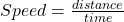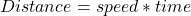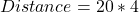## Page A car is moving at a rate of 72 km/ hr. How far does the moves stop after 4 seconds

Question

Page
A car is moving at a rate of 72 km/ hr. How far
does the moves stop after 4 seconds

in progress 0
2 months 2021-07-22T18:23:03+00:00 1 Answers 8 views 0

Distance = 80 meters

Explanation:

Given the following data;

Speed = 72 km/hr

Time = 4 seconds

Conversion:

72 km/hr to meters per seconds = 72*1000/3600 = 72000/3600 = 20 m/s

To find the distance covered by the car;

Speed can be defined as distance covered per unit time. Speed is a scalar quantity and as such it has magnitude but no direction.

Mathematically, speed is given by the formula;Making distance the subject of formula, we have;Substituting into the above formula, we have;Distance = 80 meters